# Mathematics > Naval_Mathematics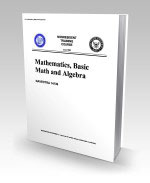14139.pdf - Pages: 304 - 14139 Mathematics, Basic Math and Algebra - Provides a review of basic arithmetic and elementary algebra; includes fractions, decimals, percentages, exponents, radicals, logarithms; exercises in factoring polynomials, linear equations, ratio, proportion, variation, complex numbers and quadratic equations; presents brief introduction to plane figures, geometric construction, and trigonometry.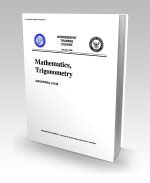14140.pdf - Pages: 272 - 14140 Mathematics, Trigonometry - Contains information on the following subjects: definitions, notations, and computations with logarithms; trigonometric ratios, analysis, applications, and aids to computations; trigonometric identities; and vectors and forces. This course is general in nature and is not directed toward any one specific specialty.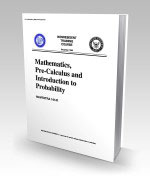14141.pdf - Pages: 384 - 14141 Mathematics, Pre-Calculus and Introduction to Probability - Contains information on the following subjects: straight lines, conic sections, tangents, normals, slopes; introduction to differential and integral calculus; combinations and permutations; and introduction to probability. This course is general in nature and is not directed toward any specific specialty.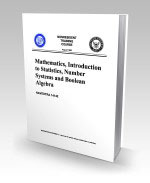14142.pdf - Pages: 226 - 14142 Mathematics, Introduction to Statistics, Number Systems and Boolean Algebra - Contains information on the following subjects: elements of digital computer mathematics--sequence and series, induction and the binomial theorem, statistics, number systems, sets and subsets, Boolean algebra, matrices, and determinants.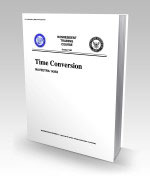14252.pdf - Pages: 50 - 14252 Time Conversion - Assists enlisted and officer personnel of the United States Navy and Naval Reserve in acquiring the knowledge requisite to the computation of time. It uses two-dimensional charts and expanded narratives to explain both the global division and designation, and the processes and mathematical formulas used in the conversion of time.